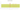# ر.ق.‏

## Qatari Rial

The official currency of the State of Qatar is the riyal of Qatar. It is denoted by the letters QR or r.q and is divisible into 100 dirhams.

#### how has the value of the currency changed in the last year?## USD 1 = QAR 3.6465

How much is10 US dollars worth inQatari rials?
At the current exchange rate, 10 US dollars is worth 36.47 Qatari rials
How much is50 US dollars worth inQatari rials?
At the current exchange rate, 50 US dollars is worth 182.33 Qatari rials
How much is100 US dollars worth inQatari rials?
At the current exchange rate, 100 US dollars is worth 364.65 Qatari rials
How much is500 US dollars worth inQatari rials?
At the current exchange rate, 500 US dollars is worth 1,823.26 Qatari rials
How much is2,000 US dollars worth inQatari rials?
At the current exchange rate, 2,000 US dollars is worth 7,293.04 Qatari rials
How much is10 US dollars worth inQatari rials?
At the current exchange rate, 10 US dollars is worth 36.47 Qatari rials
How much is50 US dollars worth inQatari rials?
At the current exchange rate, 50 US dollars is worth 182.33 Qatari rials
How much is100 US dollars worth inQatari rials?
At the current exchange rate, 100 US dollars is worth 364.65 Qatari rials
How much is500 US dollars worth inQatari rials?
At the current exchange rate, 500 US dollars is worth 1,823.26 Qatari rials
How much is2,000 US dollars worth inQatari rials?
At the current exchange rate, 2,000 US dollars is worth 7,293.04 Qatari rials